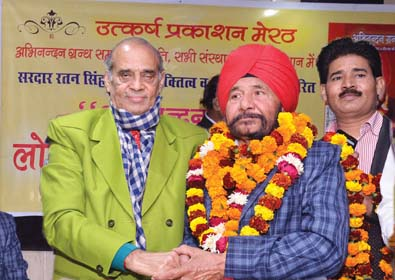Previous Nextडा. हरिओम पंवार ने किया राष्ट्रीय हास्य कवि सरदार रतन सिंह रतन की उपलब्धियों पर आधारित अभिनन्दन ग्रन्थ का लोकार्पण उत्कर्ष प्रकाशन द्वारा आयोजित यह समारोह मेरठ में संपन्न हुआ

Rs. 100 Rs. 90

Rs. 100 Rs. 90

Rs. 150 Rs. 135

Rs. 150 Rs. 135

Rs. 100 Rs. 90

Rs. 100 Rs. 90

Rs. 100 Rs. 90

Rs. 100 Rs. 90

Rs. 150 Rs. 135

Rs. 250 Rs. 225

Rs. 150 Rs. 135

Rs. 150 Rs. 135

Rs. 150 Rs. 135

Rs. 150 Rs. 135

Rs. 150 Rs. 135

Rs. 150 Rs. 135

Rs. 150 Rs. 135

Rs. 150 Rs. 135

Rs. 250 Rs. 225